Question

# Design a circuit that reads as inputs continuous bits, and generates an output of ‘1’ if...

Design a circuit that reads as inputs continuous bits, and generates an output of ‘1’ if the sequence (0011) is detected using JK Flip Flop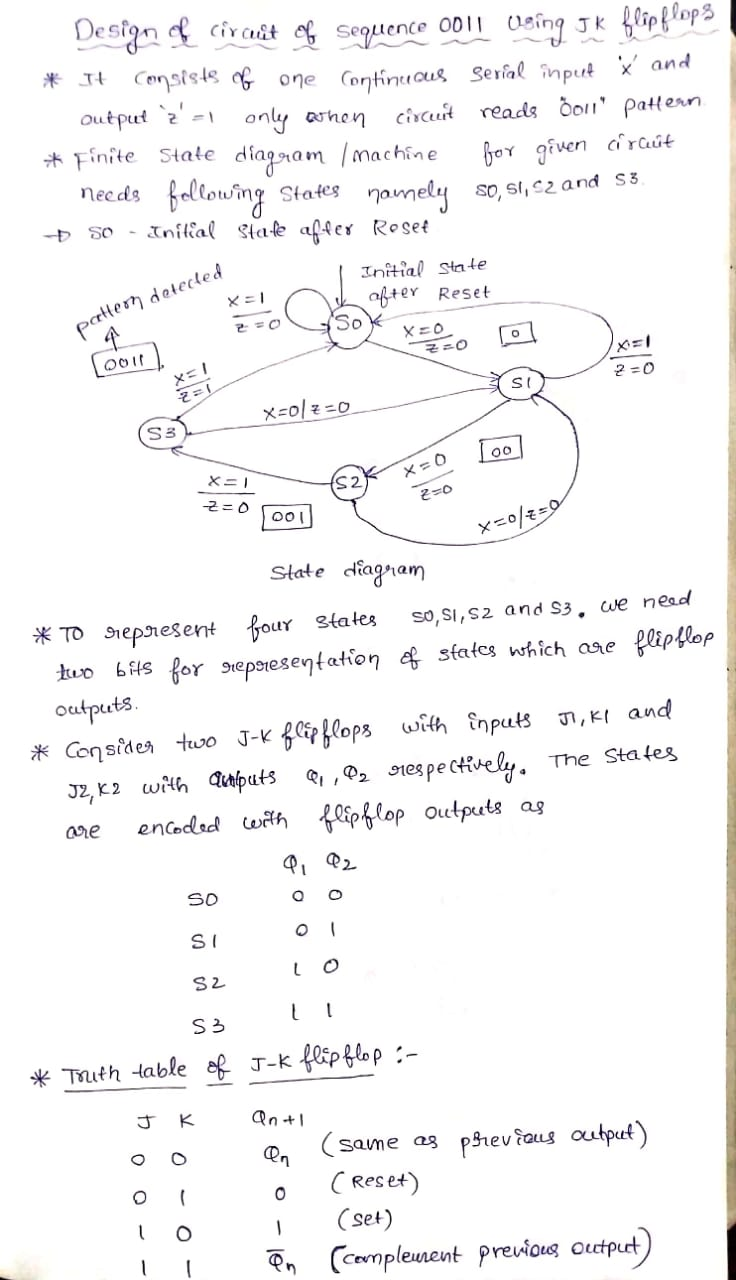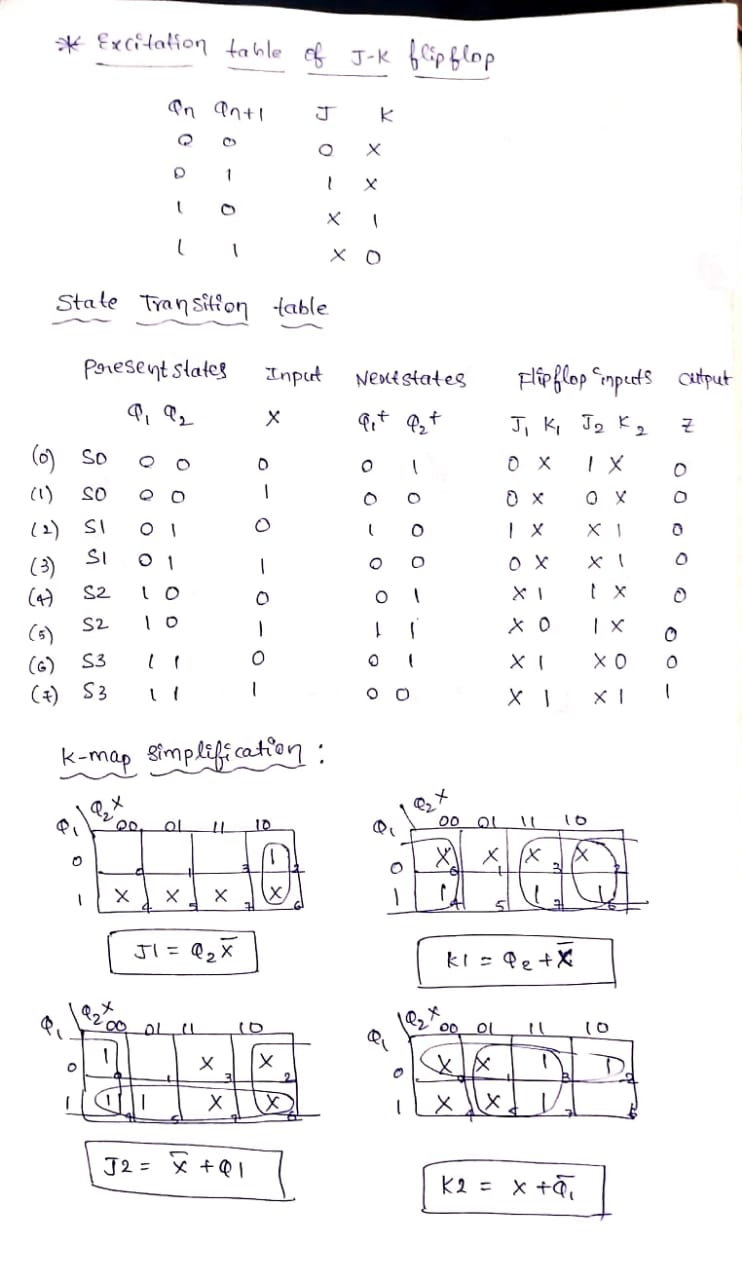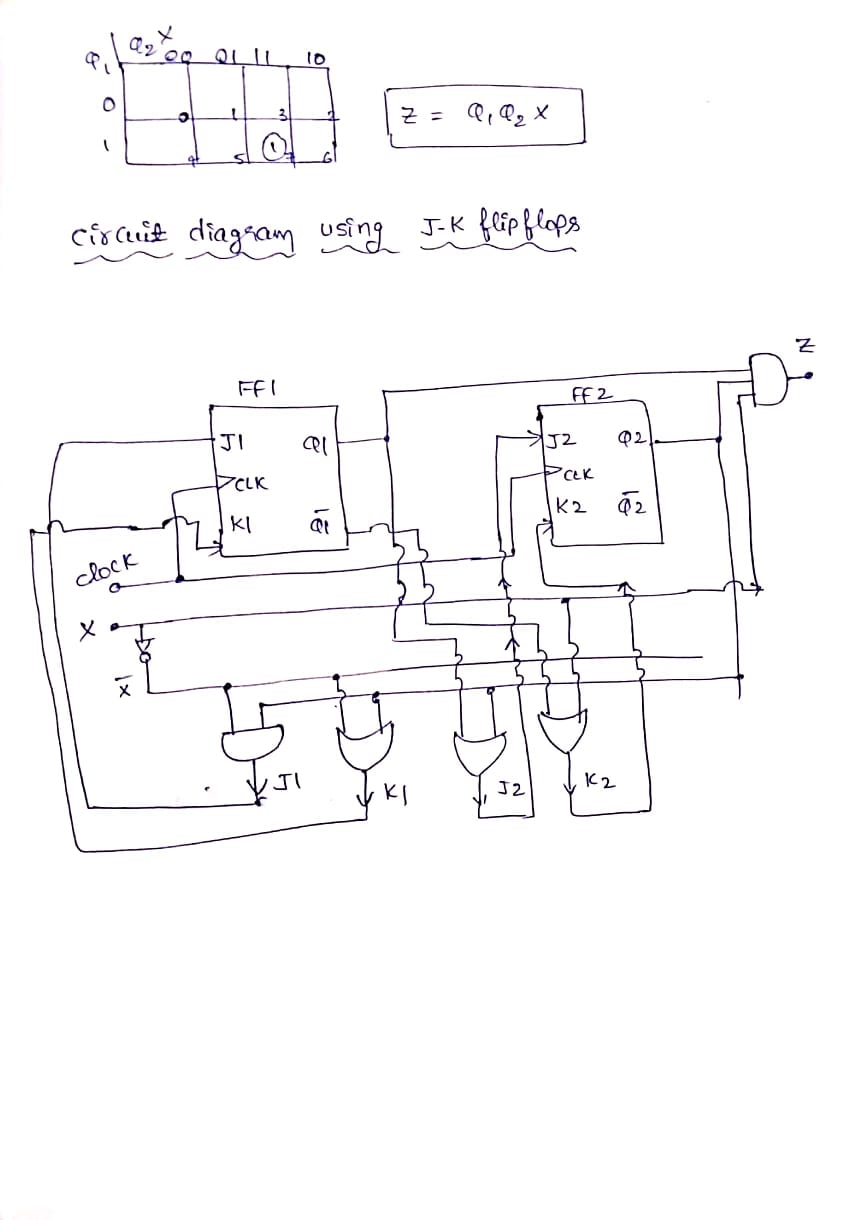#### Earn Coins

Coins can be redeemed for fabulous gifts.

Similar Homework Help Questions
• ### Question #2. Design of a Sequential Circuit: A SEQUENCE DETECTOR that detects the sequence 10 must...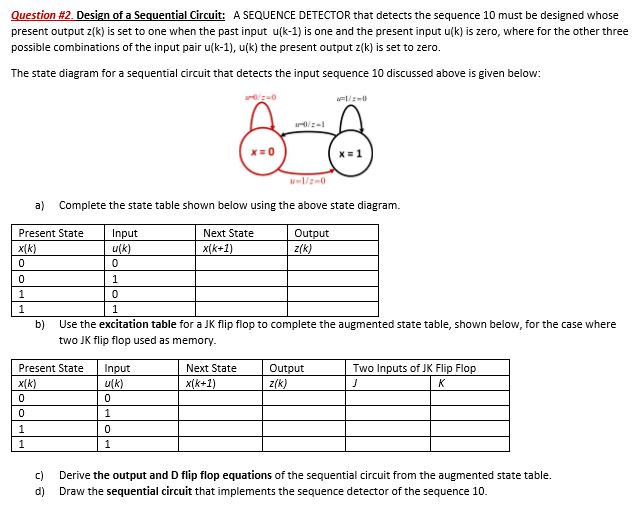Question #2. Design of a Sequential Circuit: A SEQUENCE DETECTOR that detects the sequence 10 must be designed whose present output z(k) is set to one when the past input u(k-1) is one and the present input u(k) is zero, where for the other three possible combinations of the input pair u(k-1), u(k) the present output z(k) is set to zero. The state diagram for a sequential circuit that detects the input sequence 10 discussed above is given below: AA...

• ### design a combinational circuit that input 4 bits and generates 16 bits output. Solve for the...

design a combinational circuit that input 4 bits and generates 16 bits output. Solve for the AND OR gate (Hint start by creating a truth table) i will rate you thumbs up

• ### 3) A digital circuit is shown input output input 4 input This circuit performs the function...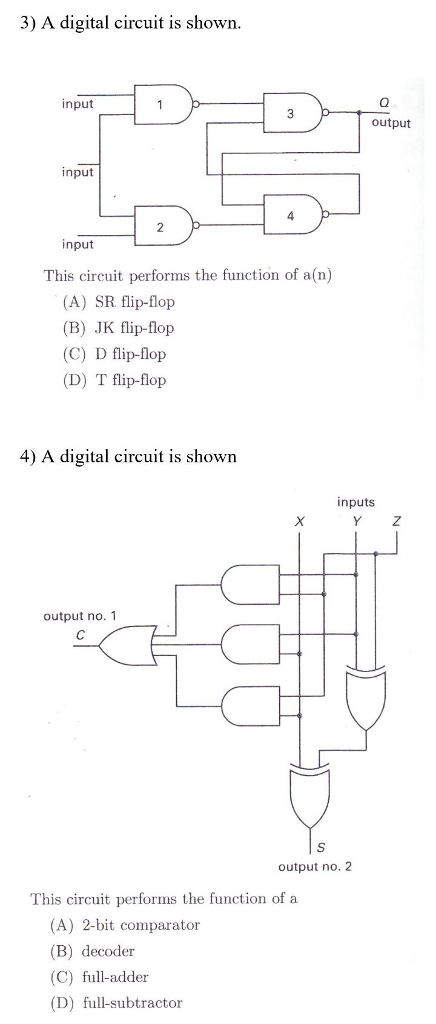3) A digital circuit is shown input output input 4 input This circuit performs the function of a(n) (A) SR flip-flop (B) JK flip-flop (C) D flip-flop (D) T flip-flop 4) A digital circuit is shown inputs Y Z output no. 1 output no. 2 This circuit performs the function of a (A) 2-bit comparator (B) decoder (C) full-adder (D) full-subtractor

• ### Question 2 [15 Ptsl Flip-flops: Using D flip-flop, design a one input, one output serial 2's...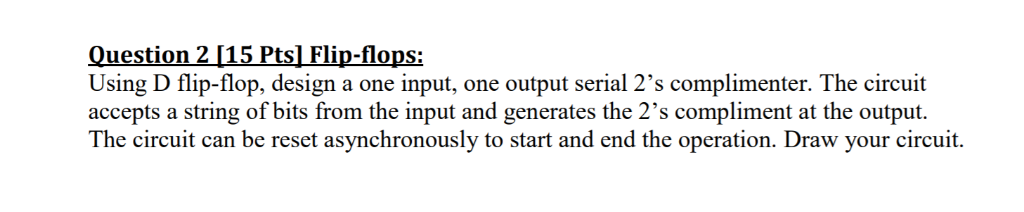Question 2 [15 Ptsl Flip-flops: Using D flip-flop, design a one input, one output serial 2's complimenter. The circuit accepts a string of bits from the input and generates the 2's compliment at the output. The circuit can be reset asynchronously to start and end the operation. Draw your circuit.

• ### 2. Design an even parity detection circuit. A parity bit is an error checking mechanism. Your...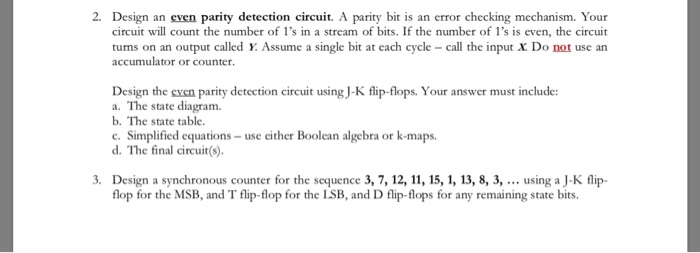2. Design an even parity detection circuit. A parity bit is an error checking mechanism. Your circuit will count the number of 1's in a stream of bits. If the number of l's is even, the circuit turns on an output called y. Assume a single bit at each cycle - call the input x. Do not use an accumulator or counter. Design the even parity detection circuit using J-K flip-flops. Your answer must include: a. The state diagram. b....

• ### Please label the circuit as well, the inputs and outputs Design a sequence detector that examines...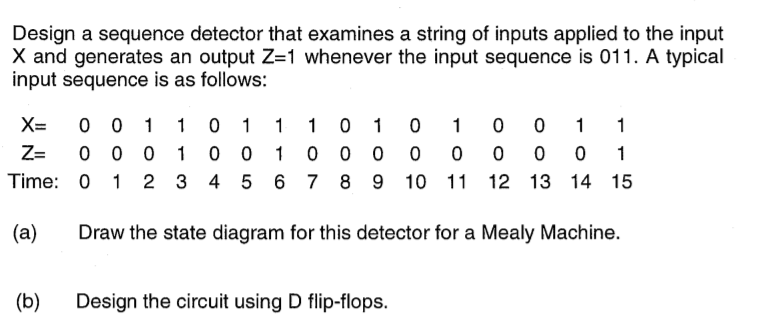Please label the circuit as well, the inputs and outputs Design a sequence detector that examines a string of inputs applied to the input X and generates an output Z-1 whenever the input sequence is 011. A typical input sequence is as follows: X 0 01 1 01 1 1 0 1 0 1 0 0 1 1 Z 0 0 01 0 01 0 0 0 0 0 0 0 0 1 Time: 0 1 2 3 4 5...

• ### 4. For the following state table 00 11 01 01 00 1 1 01 11 jus Design the system using a T flip flop for q, and an SR flip flop for the equations for the flip flop inputs and the output. 4. F...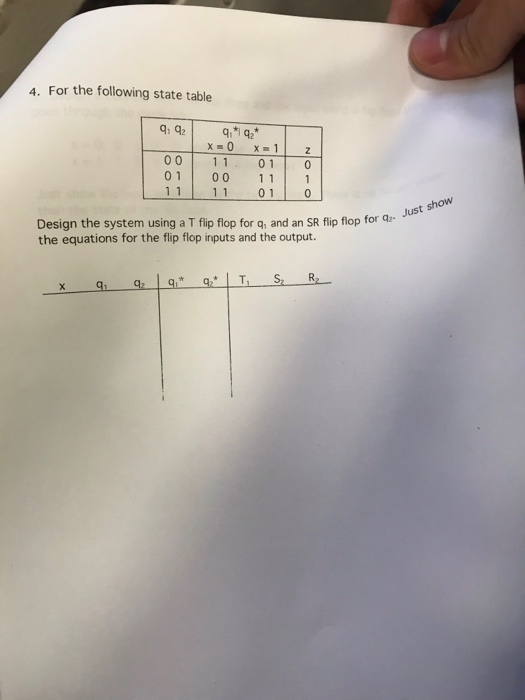4. For the following state table 00 11 01 01 00 1 1 01 11 jus Design the system using a T flip flop for q, and an SR flip flop for the equations for the flip flop inputs and the output. 4. For the following state table 00 11 01 01 00 1 1 01 11 jus Design the system using a T flip flop for q, and an SR flip flop for the equations for the flip flop...

• ### Given two inputs, E and F, two strings of bits are received. We wish to design...

Given two inputs, E and F, two strings of bits are received. We wish to design a synchronous sequential system that detects when the information received by these inputs is equal to the one received on the previous instant, giving an output Z=1 when they're identical and Z=0 if they're not. Find the Mealy machine associated with this circuit and, using T flip-flops activated by a rising signal, find the functions of the flip-flops.

• ### Q5: 1. Design the circuit of JK Flip-Flop using DFF and derive state table and characteristic...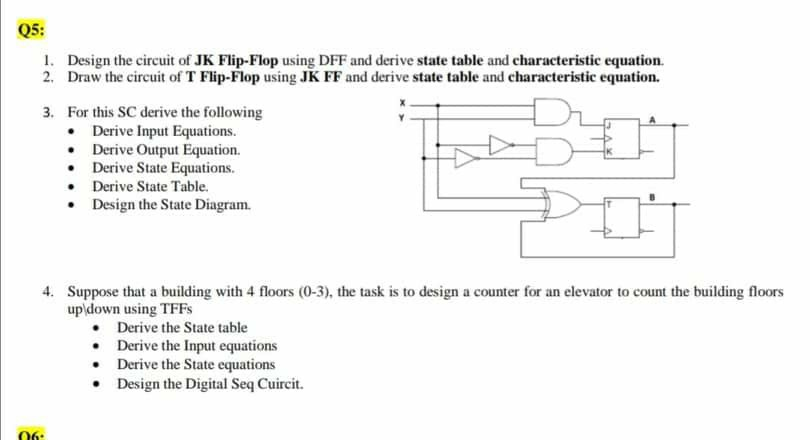Q5: 1. Design the circuit of JK Flip-Flop using DFF and derive state table and characteristic equation. 2. Draw the circuit of T Flip-Flop using JK FF and derive state table and characteristic equation. 3. For this SC derive the following • Derive Input Equations. • Derive Output Equation. • Derive State Equations. • Derive State Table • Design the State Diagram. 4. Suppose that a building with 4 floors (0-3), the task is to design a counter for an...

• ### Q5: 1. Design the circuit of JK Flip-Flop using DFF and derive state table and characteristic...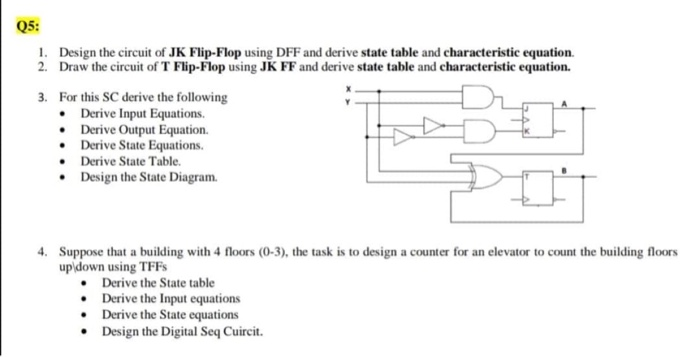Q5: 1. Design the circuit of JK Flip-Flop using DFF and derive state table and characteristic equation. 2. Draw the circuit of T Flip-Flop using JK FF and derive state table and characteristic equation. 3. For this SC derive the following • Derive Input Equations. • Derive Output Equation. • Derive State Equations. • Derive State Table • Design the State Diagram. 4. Suppose that a building with 4 floors (0-3), the task is to design a counter for an...